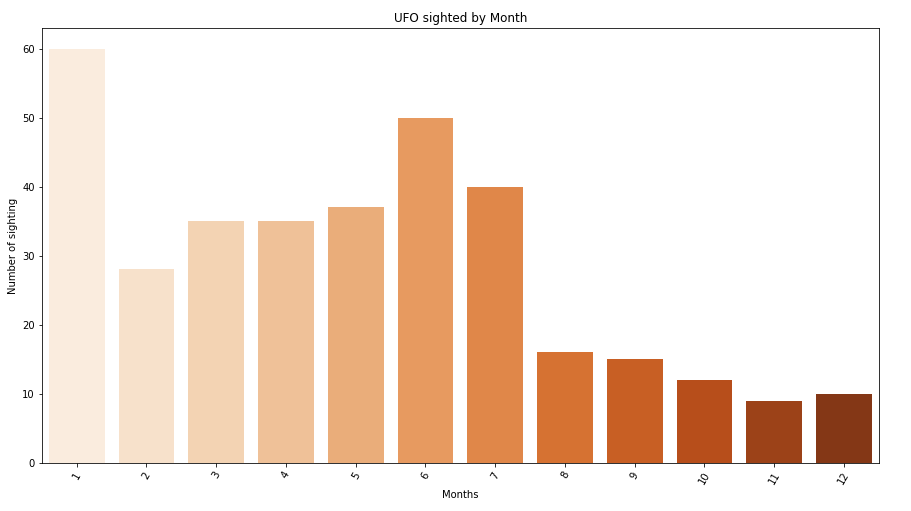﻿ Pandas Datetime: Create a graphical analysis of UFO sighted by month - w3resource

# Pandas Datetime: Create a graphical analysis of UFO sighted by month

## Pandas Datetime: Exercise-21 with Solution

Write a Pandas program to create a graphical analysis of UFO (unidentified flying object) sighted by month.

Sample Solution:

Python Code:

``````import pandas as pd
import matplotlib.pyplot as plt
import seaborn as sns
df['Date_time'] = df['Date_time'].astype('datetime64[ns]')
df["ufo_yr"] = df.Date_time.dt.month
months_data = df.ufo_yr.value_counts()
months_index = months_data.index  # x ticks
months_values = months_data.get_values()
plt.figure(figsize=(15,8))
plt.xticks(rotation = 60)
plt.title('UFO sighted by Month')
plt.xlabel("Months")
plt.ylabel("Number of sighting")
months_plot = sns.barplot(x=months_index[:60],y=months_values[:60], palette = "Oranges")
``````

Sample Output:

``````

Python Code Editor:

Have another way to solve this solution? Contribute your code (and comments) through Disqus.

What is the difficulty level of this exercise?

﻿

Inviting useful, relevant, well-written and unique guest posts